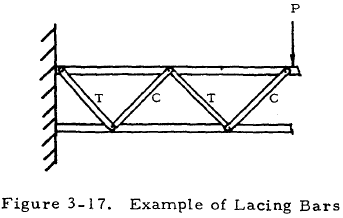﻿ Bar Analysis | Engineering Library

# Bar Analysis

This page provides the sections on bar analysis from the "Stress Analysis Manual," Air Force Flight Dynamics Laboratory, October 1986.

Other related chapters from the Air Force "Stress Analysis Manual" can be seen to the right.

Bar Analysis

## 3.1 Introduction to Bar Analysis

Bars are thin structural members. This chapter gives procedures for determining the resistance to yielding of bars under static loads as well as their resistance to fatigue failure under varying loads. Tensile loading of bars is considered in detail, and information applicable to the compressive, bending, and torsional loading of bars appearing in other chapters is referenced in this chapter.

## 3.2 Nomenclature for Bar Analysis

 A = cross-sectional area, in2 ft = tensile stress, psi Fty = yield stress in tension, psi K = stress concentration factor Ke = effective stress concentration factor Kt = theoretical stress concentration factor
 n = factor of safety P = load, lbs q = notch sensitivity factor

The basic formula for stress in a member of cross-sectional area A under a static tensile load P is

$$f_t = { P \over A }$$
(3-1)

This equation, however, is somewhat limited. In order for it to be valid, the member must be centrally loaded, the section at which σ occurs must be well removed from the point of application of the load, and no stress raisers may be present near the section where σ occurs.

Bars are normally designed so that tensile loads are applied centrally. If this is not the case, they may be considered to be beams under combined tensile and bending loads and treated with the material in Chapter 1. Although the end portions of a bar are as critical as the central ones, they are not considered here since information about them more properly belongs in a treatment of connections, for example, the chapter on lug analysis in this work. According to St. Venant's principle, stress patterns become regular at a distance from the point of application of a load. In this case, the stresses become uniform at a distance from the point of application of an axially applied tensile load. Stress raisers will be considered here and when they are present, Equation (3-1) no longer applies.

If a stress raiser is present in a bar loaded in tension,

$$f_t = K \left({ P \over A }\right)$$
(3-2)

where K is a stress concentration factor. Equation (3-2) indicates that the stress at the discontinuity is K times the stress that would occur if no stress raiser were present. The stress concentration factor may be determined theoretically by the theory of elasticity, the photoelasticity method, etc., where it is designated as Kt. These values are not normally accurate, however, and are not in general used directly as will be discussed later. The following figures give values of the theoretical stress concentration factor for various cross-section and discontinuity shapes.

The theoretical stress concentration factor may be quite high as can be seen in the following pages; however, this value is usually in agreement with experiment. To account for this discrepancy, an effective stress concentration factor Ke is defined to be the one that holds in the actual situation encountered. The notch sensitivity factor, q, is used to relate the two and is defined by Equation (3-3):

$$q = { K_e - 1 \over K_t - 1 }$$
(3-3)

The value of the notch sensitivity factor is a function of the material and the size and shape of the discontinuity.

For ductile materials that are statically loaded to near their limit, the yielding in the vicinity of the discontinuity may nearly eliminate stress concentration there, so that Ke is approximately equal to one and q is quite low.

Brittle homogeneous materials are not as capable of localized yielding, so that Ke is approximately equal to Kt and consequently q is approximately equal to one according to Equation (3-3). Cast iron of less than 45, with its flakes of graphite, however, is effectively saturated with stress raisers, so that the addition of another discontinuity seems to have little effect on its fatigue strength. Thus q is approximately equal to zero for cast iron.

The design equation for a bar under a static tensile load P that is not to be subjected to large-scale yielding is thus

$$F_{ty} = K_e \left({ P \over A }\right)$$
(3-4)

For a ductile or cast iron bar, Ke may be assumed to be equal to unity and A is the reduced area at the section where the discontinuity occurs. For a bar of brittle homogeneous material, Ke may be assumed to be equal to Kt and A is either the cross-sectional area of the bar without the discontinuity or the reduced area at the cross section where the discontinuity occurs. Which of these areas is to be used is shown in a formula under each chart of Kt in Figures 3-1 through 3-12.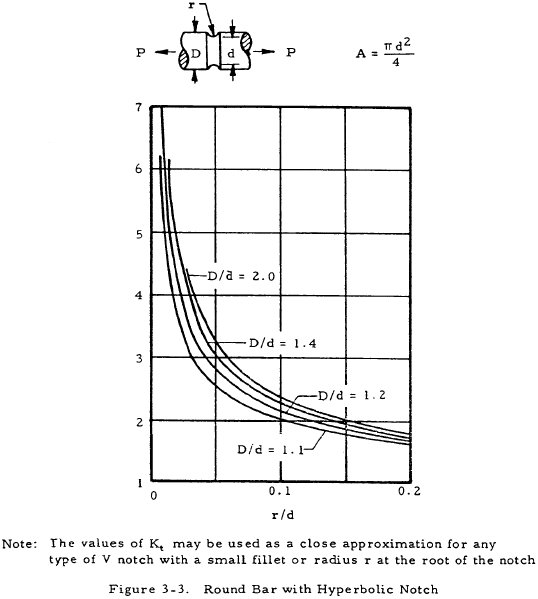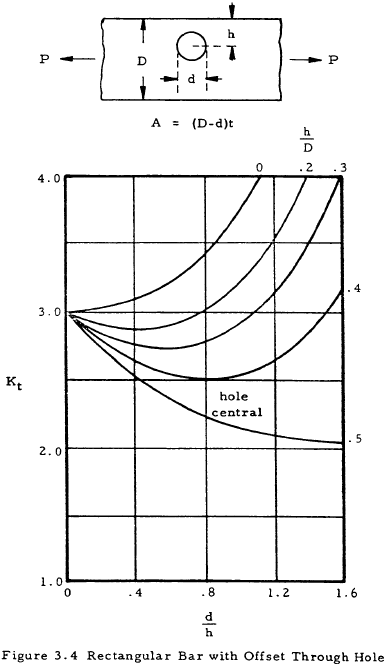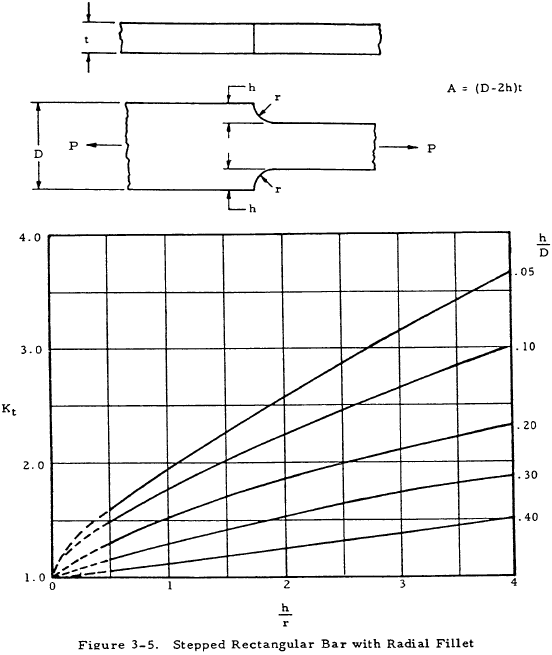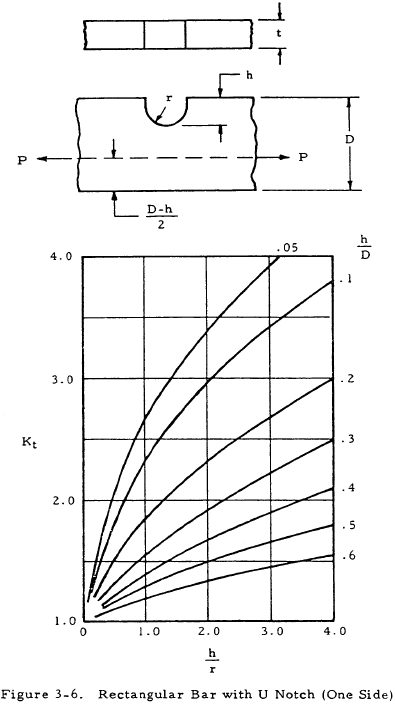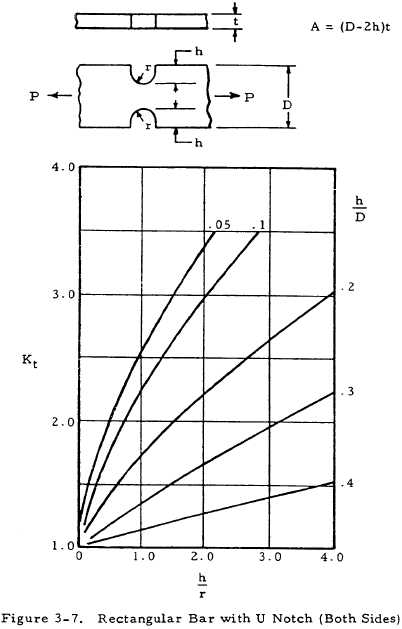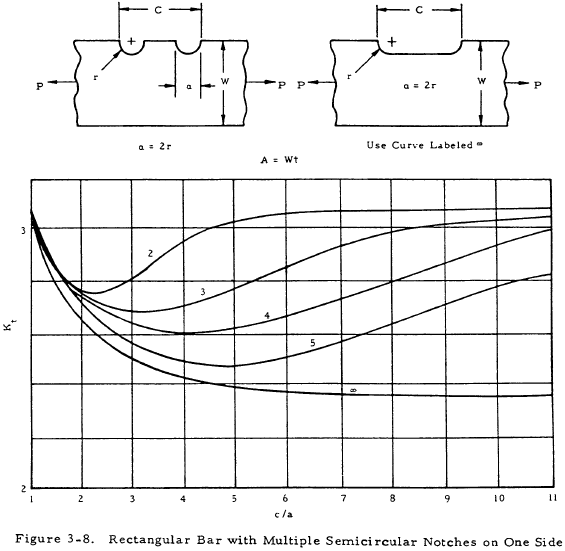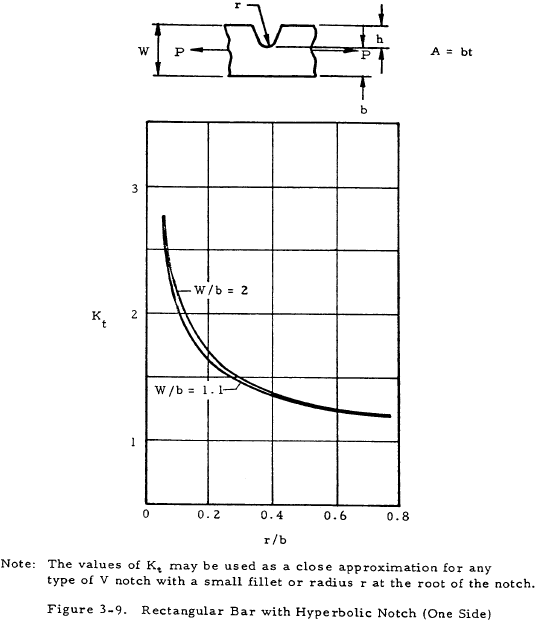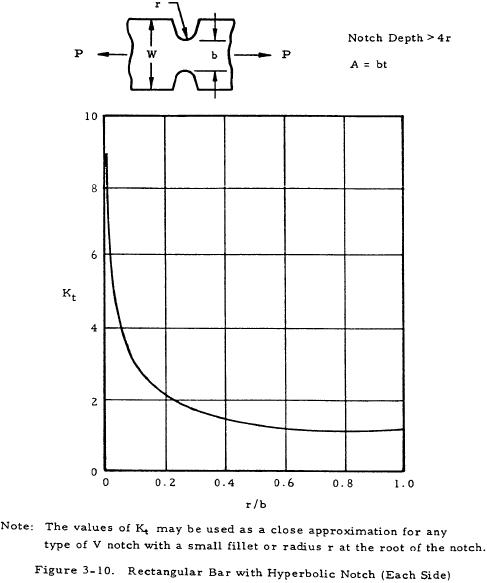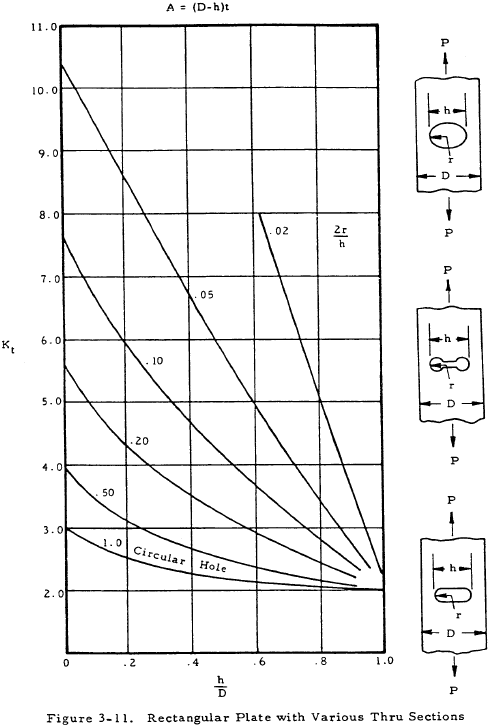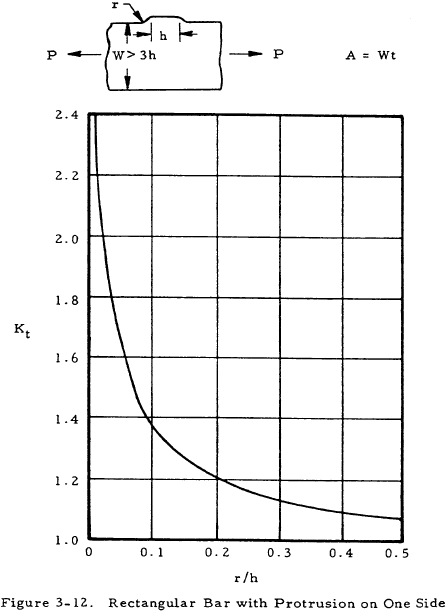The previously described procedure yields conservative results for brittle homogeneous materials and the opposite for cast iron and ductile materials. If a low margin of safety is desired for ductile materials and cast iron, a value of q equal to 0.2 is usually sufficient to account for the effects of stress raisers.

The previously discussed material on bars in static tensile loading is summarized in Table 3-1.

Table 3-1: Design of Bars Under Static Tensile Load

Design Equation $$F_{ty} = K_e \left({P \over A}\right)$$

Material Type Ke A
ductile 1.0 reduced area of cross section at the discontinuity
homogeneous brittle Kt as found in Figures 3-1 thru 3-12 either reduced or total area of cross section as indicated by formulas in Figures 3-1 thru 3-12.
cast iron 1.0 reduced area of cross section at the discontinuity

We have a number of structural calculators to choose from. Here are just a few:

## 3.4 Sample Problem - Bar Under Static Tensile Loads

Given: A circular bar is to be made of a homogeneous brittle material for which Fty = 45,000 psi. It is to support a static tensile load of 50,000 lbs with a factor of safety of 1.5. It is to have a U-notch of the section shown in Figure 3-13.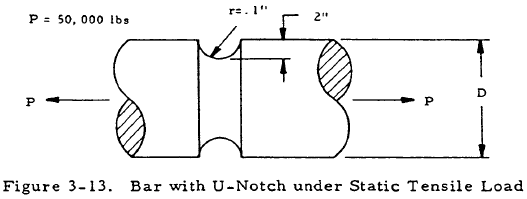Find: The diameter

Solution: Inserting the safety factor into Equation (3-4) gives

$${ F_{ty} \over 1.5 } = { 45000 \over 1.5 } = K_e { P \over A } = 30,000$$

From Section 3.3, Ke = Kt. Thus,

$$30,000 = K_t { P \over A }$$

Since P = 50,000, $$0.6 = { K_t \over A }$$.

From Figure 3-2, h = 0.2, r = 0.1 thus, h/r = 2.

And furthermore,

$$A = { \pi (D - 0.4)^2 \over 4 }$$

Thus,

$$0.6 = { 4 ~K_t \over \pi (D - 0.4)^2 }$$

Assume D = 1.05. Thus,

$${ h \over D } = { 0.2 \over 1.05 } = 0.190$$

From Figure 3-2, Kt = 2.0. Substituting these values gives

$${ 4 K_t \over \pi (D - 0.4)^2 } = { 4 (2.0) \over \pi (1.05 - 0.4)^2 } = 0.6$$

Thus, D is equal to 1.05 in. If the assumed value of D does not satisfy the equation, other values must be tried until a value of D satisfying this equation is found.

Bars that are subjected to compressive loads may be considered to be columns. The behavior of columns may be obtained by referring to Chapter 2.

## 3.8 Bending Loads on Bars

Bars that are subjected to bending loads may be considered to be beams and treated with the material on beams in bending appearing in Chapter 1.